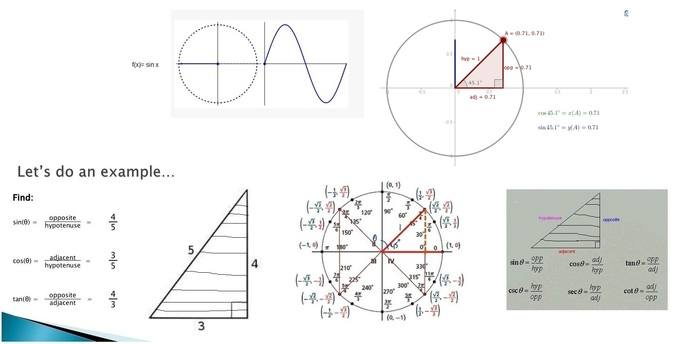# Few basic trigonometry questions ?(the x , after the sin is the representation of angle in theta) and it also means ( opposite side length/ hypotenuse side length )

how are these things related ? i mean the angle in theta , the opposite side length / hypotenuse side length ... ??

mfb
Mentor
For a given angle theta, the ratio of those two sides is always the same, no matter how large the triangle is. The relation between the two is the sine function by definition.

thanks ,

if you take a sin x , which is sin theta ... it means ... opposite side length / hypotenuse side length ...

the change in the angle is basically going to have no effects at all on the length of the sides of the right angled triangle ... right ?

also at the same time the ratio simply means ,opposite side length / hypotenuse side length ... right ?

for example ,

if you take sin x , and you are given the measurement of the sides of the right angled triangle ...

it again simply means , sin x is opposite side length / hypotenuse side length ...

my problem was , i have always seen this like some sort of propagation of values ...

i still don't know why i see this ratio , opposite side length / hypotenuse side length like some sort of value that propagates ...

it is simply a constant value , or ratio of the outer lengths of the right angled triangle depending on the sine , cosine or tan function ??

which is also the ,

sin x , opposite side length/ hypotenuse side length
cos x , adjacent side length / hypotenuse side length
tan x , opposite side length / adjacent side length

??

Last edited by a moderator:
mfb
Mentor
the change in the angle is basically going to have no effects at all on the length of the sides of the right angled triangle ... right ?
Which change? If you change the angle, the ratio changes as well.
also at the same time the ratio simply means ,opposite side length / hypotenuse side length ... right ?
Yes, that's the ratio we are talking about.
i have always seen this like some sort of propagation of values
What does that mean?
it is simply a constant value , or ratio of the outer lengths of the right angled triangle depending on the sine , cosine or tan function ??
I don't understand that question.
sin x , opposite side length/ hypotenuse side length
cos x , adjacent side length / hypotenuse side length
tan x , opposite side length / adjacent side length
That is the definition of the functions sine, cosine and tangent. Well, one of many possible equivalent definitions.

Do you understand what a function is?

thanks for the answers ... i have few more questions ...

i am really trying to understand a few things from the basics ...

i don't really understand what the word ratio , means in a triangle ....

are we trying to find the length of that line passing between the

sin x , opposite side length/ hypotenuse side length
cos x , adjacent side length / hypotenuse side length
tan x , opposite side length / adjacent side length

???

Last edited by a moderator:
mfb
Mentor